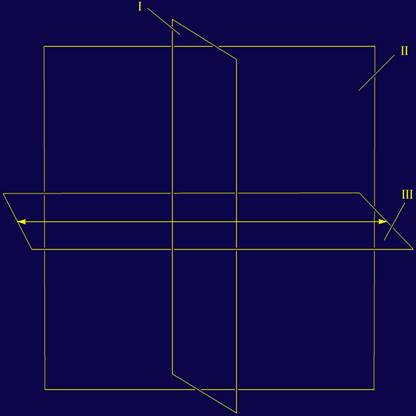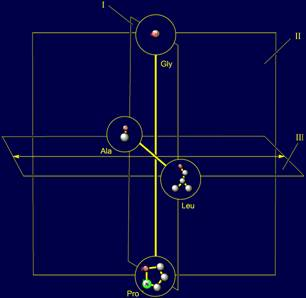5. Variant of the construction of canonical amino acids system without a dodecahedron

5.1. Construction of system of 20 elements without a dodecahedron

There is a possibility of construction of system of amino acids without use of a dodecahedron. We offer a variant of such a system of 20 elements. In principle, the entire procedure repeat the version of the construction on the dodecahedron. Someone may like this version more. Figure 23 shows the partition of the three planes of antisymmetry.

.

 Fig. 23. Planes of antisymmetry for construction of system of 20 elements without the dodecahedron.   I - a plane of quasi-mirror antisymmetry; II - a plane of not mirror antisymmetry; III - a plane of rotary antisymmetry.The sequence of construction of system of 20 elements without the dodecahedron is shown in figure 24.

 Fig. 24. Stages of construction of system of 20 elements without the dodecahedron. a - elements A, B, –A, –B in the plane I; b - symmetric elements of type A on a vertical parallelepiped; c - symmetric elements of type B on a horizontal parallelepiped; d - a complete  system of 20 elements without the dodecahedron.

5.2. Construction of system of 20 side chains of amino acids without the dodecahedron

We also examined a variant of an arrangement of system of the side chains of amino acids without the dodecahedron. Consecutive stages of construction of this system are resulted without comments on figure 25, a-d.a Fig. 25. Consecutive stages of construction of complete system of 20 amino acids without the dodecahedron. a - amino acids of 1 and 2 subgroups; b - amino acids of 3 subgroups on a vertical parallelepiped; c - amino acids of 4 subgroups on a vertical parallelepiped;  d - complete system of four subgroups of amino acids.

The system of amino acids without the dodecahedron also consists of four subgroups which can be connected by transformations of alpha, beta and gamma types and their combinations. However, in contrast to the dodecahedron, it loses one important property: the relationship with the dodecahedron, ensuring the integrity of the canonical set of amino acids. In more details these questions will be discussed on our page: http://vector-machine.narod.ru/index_e.htm

Адрес для связи: amino-acids-20@yandex.ru

Сайт управляется системой uCoz